# Equivalence of random coinflips view and minimal programs view

Two of the several ways of viewing the deterministic variant of Solomonoff induction are:

• The "random coinflips view": Given a monotone Turing machine, we feed the machine with random data (such as a string produced from unbiased coinflips). The probability of string$x$ is then the probability that the machine outputs something starting with$x$. This one is somewhat hard to think about, because one usually thinks of a Turing machine as e.g. a Python interpreter that takes Python programs. If one feeds a Python interpreter with randomness, one is likely to end up running a horrible mess that isn't even syntactically valid. I guess the reply here is something like (1) if a program isn't even syntactically valid, it just results in a program with no output; (2) using an encoding, one can filter out all invalid programs so that each input into the UTM does something; (3) more generally, thinking of "coinflips" is meant to suggest the idea that one has no information about what the program looks like, so the thinking is something like "ok, this machine is running some program but I have no idea what it is; it might as well come from random coinflips". (There is a misconception one might have in that in the real world, if one is presented with a computer running "some program", one usually has some "background information", e.g. that certain programs are commonly run utility software or are lucrative to run (e.g. bitcoin mining), hence have more weight placed on them. But in this abstract setting, one is not allowed to use this background information.)
• The "minimal programs view": Given a monotone Turing machine$T$, the minimal programs producing$x$ (a.k.a. "minimal for$x$") are those programs$p$ such that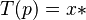$T(p) = x*$ (i.e.$T$ when run with$p$ produces an output starting with$x$) and such that no proper prefix of$p$ produces$x$ (i.e. if$q \prec p$ then$T(q) \ne x*$). This view then says that to get the probability of$x$, we add up all the minimal programs weighted by length as follows: for each program$p$ we assign the weight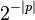$2^{-|p|}$, where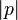$|p|$ is the length of$p$ in bits. Thus the measure is${\textstyle \sum_p 2^{-|p|}}$, where the sum is taken over all programs$p$ which are minimal for$x$. The idea with the$2^{-|p|}$ is that there are$2^n$ programs of length$n$, so given just the information that$p$ has length$n$, the probability that we have a specific program is$1/2^n = 2^{-n}$.

These two views turn out to be equivalent in the sense that they assign exactly the same probability to every string.

## Is this result obvious?

Having the details spelled out, one might believe the equivalence is obvious. I will quote the following, which I wrote down on 2019-03-25, as evidence that this equivalence is not obvious: "It seems to me like the 'random coinflips' probability of getting a string starting with x is not the same as the sum of the probabilities of the minimal programs that print a string starting with x. And yet, everyone seems to equate the two. My intuition is that the minimal programs one ignores programs that print x but aren't minimal, whereas the random coinflips one takes these into account." (My confusion here is that the coinflips one also ignores certain programs, but it just ignores different programs.)

## Example

Let$T$ be a monotone Turing machine whose behavior is as follows. Note that$T$ isn't a universal machine, so this example isn't actually an instance of the equivalence, but is still illustrative and gives a feel for how these things work.

Line Input Behavior
1 0 x
2 1$\epsilon$ (empty string)
3 10 xy, halts
4 11$\epsilon$, halts
5 01 x, halts
6 00 x, halts

We can also implement$T$ as an interactive algorithm as follows:

def T:
b := input()
if b == 0:
print("x")
b := input()
else:
b := input()
if b == 0:
print("xy")


The above table exactly captures the behavior for$T$ since all four length-2 strings lead to a halting state,$T$ does nothing more when fed a longer input.

One can verify that$T$ is monotone: 0 is a prefix for 01 and 00, and indeed x is a prefix for x and x. Similarly, 1 is a prefix for 10 and 11, and indeed$\epsilon$ is a prefix for$\epsilon$ and xy.

Now let us see what probability each "view" assigns to the output starting with x.

• Random coinflips view: the idea is to generate an infinite sequence of coinflips, e.g. 1010010110100001... and feed this into$T$. But since our machine does nothing more after two bits are fed in, we can get away with flipping the coin just twice. So our possible coinflip outcomes are 00, 01, 10, 11, each with probability 1/4. Given these cases, in three of them we end up with an output starting with x (lines 3, 5, 6 in the table above). So the probability is 3/4.
• Minimal programs view: the minimal programs that produce x are 0 (line 1) and 10 (line 3). Note that programs 01 and 00 are not minimal for x because a shorter program (namely 0) also already produces x. So according to this view, the probability is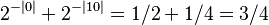$2^{-|0|} + 2^{-|10|} = 1/2 + 1/4 = 3/4$. (Note that$|0|=1$ is the length of the program 0, not the absolute value of the number 0.) Notice also that the set of programs we are adding up is prefix-free, i.e.$\{0,10\}$ is a prefix-free set.

In each case, we end up with the same probability, namely 3/4. This is despite the fact that we are adding different lines. In the coinflips view, we added lines 3, 5, 6, whereas in the in the minimal programs view we added lines 1 and 3. The equivalence "random coinflips view" = "minimal programs view" is the statement that ending up with the same probability through these different methods is not a coincidence.

## Example 2

Let$T$ be a monotone Turing machine whose behavior is as follows.

Line Input Behavior
1 0 x
2 1 halts
3 00 x, halts
4 01 xy, halts

The table specifies the behavior in all cases since 1, 00, 01 covers all ways in which the input could begin, and the machine halts in all of them (so for longer input, it produces no more output).

Let us find the prior probability that the machine outputs something starting with x.

• random coinflips view: 1/4 + 1/4 = 1/2 (add lines 3, 4)
• minimal programs view: 1/2 (line 1)

A misconception I had when I first thought up this example is that I confused "is minimal" and "is minimal for x". The program 01 (line 4) is minimal for xy but it isn't minimal for x. Having this mistake would lead to adding up 1/2 + 1/4 = 3/4 (lines 1, 4). Instead, one must specifically search for programs that are minimal for the desired output, rather than just minimal at all. A hint that something is not right with my misconception is that the set$\{0,01\}$ is not prefix-free.

## Example 3

Give a counterexample to the equivalence if we don't assume a monotone machine.

## Proof of equivalence

The probability of getting an output starting with$x$ using random coinflips is$\lambda(\{p : T(p) = x*\})$, where$\lambda$ is the uniform measure. The idea here is that we are collecting all the programs that produce$x$, and then finding its measure with respect to the uniform (completely random) measure. The trick is to realize that if$p$ outputs something starting with$x$, then since$T$ is a monotone machine, any extension of$p$ will also output something starting with$x$. To formally state this, we can use cylinder sets: if$T(p) = x*$, then for all$q \in \Gamma_p$, we have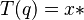$T(q) = x*$. Thus, we can say$\{p : T(p) = x*\} = \bigcup_{p : T(p)=x*} \Gamma_p$.

The weighted sum of the minimal programs for$x$ is$\sum_{p : T(p) = x*; \; p\text{ min for }x} 2^{-|p|}$. Since programs minimal for$x$ form a prefix-free set, this means that if$p,q$ are two distinct programs, neither is a prefix of the other, so the cylinder sets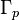$\Gamma_p$ and$\Gamma_q$ are disjoint. Since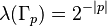$\lambda(\Gamma_p) = 2^{-|p|}$ by definition, we can thus write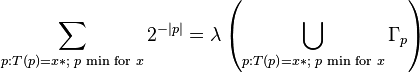$\sum_{p : T(p) = x*; \; p\text{ min for }x} 2^{-|p|} = \lambda\left(\bigcup_{p : T(p) = x*; \; p\text{ min for }x} \Gamma_p\right)$ using the fact that a measure is additive.

Thus, if we can show that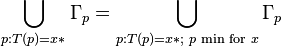$\bigcup_{p : T(p)=x*} \Gamma_p = \bigcup_{p : T(p) = x*; \; p\text{ min for }x} \Gamma_p$, this would complete the proof.

If a program produces$x$ and is minimal for$x$, then clearly it produces$x$, so the inclusion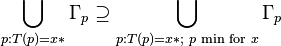$\bigcup_{p : T(p)=x*} \Gamma_p \supseteq \bigcup_{p : T(p) = x*; \; p\text{ min for }x} \Gamma_p$ is immediate.

Now let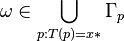$\omega \in \bigcup_{p : T(p)=x*} \Gamma_p$ (remember, each element of a cyclinder set is an infinite sequence). Then there exists a$p$ such that$T(p) = x*$ and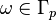$\omega \in \Gamma_p$. Now we must find a program$q$ minimal for x such that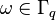$\omega \in \Gamma_q$. If$p$ is minimal for x, we are done. Otherwise there is some minimal q such that$T(q) = x*$. But we must have$q \prec p$ so that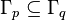$\Gamma_p \subseteq \Gamma_q$, and hence$\omega \in \Gamma_q$ as required.